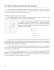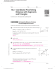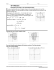Reteach Sec 1

Transcription

Reteach Sec 1
Name ________________________________________ Date __________________ Class__________________
LESSON
1-6
Reteach
Midpoint and Distance in the Coordinate Plane
The midpoint of a line segment separates the segment into two halves.
You can use the Midpoint Formula to find the midpoint of the segment
with endpoints G(1, 2) and H(7, 6).
⎛ x + x2 y1 + y 2 ⎞
⎛ 1+ 7 2 + 6 ⎞
,
,
=M⎜
M⎜ 1
⎟
2 ⎠
2 ⎟⎠
⎝ 2
⎝ 2
M is the midpoint
of HG .
⎛8 8⎞
= M⎜ , ⎟
⎝2 2⎠
= M(4, 4)
Find the coordinates of the midpoint of each segment.
1.
2.
_________________________________________
________________________________________
3. QR with endpoints Q(0, 5) and R(6, 7) __________
4. JK with endpoints J(1, –4) and K(9, 3) __________
Suppose M(3, −1) is the midpoint of CD and C has coordinates (1, 4). You can use
the Midpoint Formula to find the coordinates of D.
⎛ x + x2 y1 + y 2 ⎞
M (3, − 1) = M ⎜ 1
,
2 ⎟⎠
⎝ 2
x-coordinate of D
y-coordinate of D
3=
x1 + x2
2
Set the coordinates equal.
−1 =
y1 + y 2
2
3=
1 + x2
2
Replace (x1, y1) with (1, 4).
−1 =
4 + y2
2
6 = 1 + x2
Multiply both sides by 2.
−2 = 4 + y2
5 = x2
Subtract to solve for x2 and y2.
−6 = y2
The coordinates of D are (5, −6).
5. M(−3, 2) is the midpoint of RS , and R has coordinates (6, 0).
What are the coordinates of S?
_______________
6. M(7, 1) is the midpoint of WX , and X has coordinates (−1, 5).
What are the coordinates of W ?
_______________
Original content Copyright © by Holt McDougal. Additions and changes to the original content are the responsibility of the instructor.
1-46
Holt Geometry
Name ________________________________________ Date __________________ Class__________________
Reteach
LESSON
1-6
Midpoint and Distance in the Coordinate Plane continued
The Distance Formula can be used to find the distance d
between points A and B in the coordinate plane.
( x2 − x1 )2 + ( y 2 − y1 )2
d=
=
(7 − 1)2 + (6 − 2)2
(x1, y1) = (1, 2); (x2, y2) = (7, 6)
=
62 + 42
Subtract.
=
36 + 16
Square 6 and 4.
=
52
≈ 7.2
The distance d
between points
A and B is the
length of AB .
Use a calculator.
Use the Distance Formula to find the length of each segment or the distance
between each pair of points. Round to the nearest tenth.
7. QR with endpoints Q(2, 4) and R(−3, 9)
8. EF with endpoints E(−8, 1) and F(1, 1)
_________________________________________
9. T(8, −3) and U(5, 5)
________________________________________
10. N(4, −2) and P(−7, 1)
_________________________________________
________________________________________
You can also use the Pythagorean Theorem to find distances in the coordinate plane.
Find the distance between J and K.
c 2 = a2 + b2
2
2
Pythagorean Theorem
=5 +6
a = 5 units and b = 6 units
= 25 + 36
Square 5 and 6.
= 61
c =
Side b is
6 units.
Side a is
5 units.
Take the square root.
Use the Pythagorean Theorem to find the distance, to the nearest
tenth, between each pair of points.
11.
12.
_________________________________________
________________________________________
Original content Copyright © by Holt McDougal. Additions and changes to the original content are the responsibility of the instructor.
1-47
Holt Geometry
2. (−a, −b)
4.
3. (2d, 2e)
2. d = (2 − ( −1))2 + (2 − ( −2))2
rectangle
5. 13.4 m
6. 10 m2
7. 11.7 m
8. (17, 3)
= (3)2 + (4)2
2. (−1, −1)
3. (3, 6)
4. (5, −0.5)
5. (−12, 4)
6. (15, −3)
7. 7.1 units
8. 9 units
9. 8.5 units
10. 11.4 units
11. 5.7 units
12. 9.4 units
9 + 16
=
25
=5
4. a = 4, b = 3
c2 = 42 + 32
Reteach
1. (1, 5)
=
= 16 + 9
= 25
c=
25
=5
5. Sample answer: The Distance Formula
uses a coordinate plane. The
Pythagorean Theorem uses known
measures of two sides of a triangle.
Challenge
1. 29.6 units
LESSON 1-7
2. (−3, 2.5), (1.5, −0.5), (−0.5, 5)
3. 14.8 units
4. The perimeter of UABC is twice the
perimeter of the second triangle.
5. 12.2; 16.1 units
6. Both midpoints are at (1, 1). This is the
point where the diagonals intersect.
7. (1, 19)
8. 78.7 units; 493.2 units2
9. The diameter of the circle is
approximately 25.1 units, so the radius is
half that distance, or about 12.55 units.
The distance from the center of the circle
to G is 18 units. So G is not a point on the
circle.
Practice A
1. transformation
2. original; image
3. reflection
4. slide
5. rotation
6. 2; ABCD → A′B′C′D′
7. 3; UPQR → UP′Q′R′
8. 1; UHIJ → UH′I′J′
9.
reflection
10.
Problem Solving
1. 82.5 ft
2. 85.9 ft
3. 47.4 m
4. 18.4 m
5. B
6. H
7. C
Practice B
1. (2, 2); (−1, −2)
1. 2
2. 1
3. 3
4. rotation
Original content Copyright © by Holt McDougal. Additions and changes to the original content are the responsibility of the instructor.
A7
Holt Geometry

The Midpoint Formula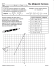Solve the problems below to answer the riddle: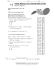Chapter One Review (Purple review worksheet)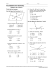Geometry Worksheet Name: Hodge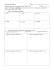STUDY GUIDE for Unit 1 Test Geometry 1. Z?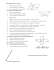Geometry Secondary Education MAFS.912.G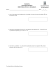Coordinate Geometry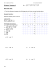1-2 Practice A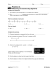Lesson 12: Dividing Segments Proportionately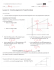Algebra with shapes How to . . .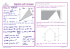Reteach Sec 1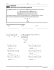HSM12CC_GM_06_09_CM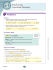5.1 Midsegment Theorem and Coordinate Proof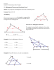Page 1 Proving a triangle is a right triangle Method 1: Show two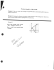HSM12CC_GM_06_08_CM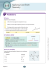8.4 The Distance Between Points